# Approximation Methods for Analysis and Formation of Mechanisms for Regulating Heat and Mass Transfer Processes in Heat Equipment Systems

Approximation Methods for Analysis and Formation of Mechanisms for Regulating Heat and Mass Transfer Processes in Heat Equipment Systems
South Ural State University, 76 Prospekt Lenina, Chelyabinsk 454080, Russia

Corresponding Author Email:
aliukovsv@susu.ru
Page:
45-58
|
DOI:
https://doi.org/10.18280/ijht.380106
9 October 2019
|
Accepted:
22 January 2020
|
Published:
31 March 2020
| Citation

OPEN ACCESS

Abstract:

The need to increase the accuracy of decisions and the depth of analysis of complex heat power systems determines the relevance of modeling and regulation of heat and mass transfer processes with the development of theoretical models and the development of a new methodology. Uncertainty of the environment increases the significance of the problems of insufficient efficiency of the available numerical approaches to the modeling of dynamic processes and the regulation of heating elements. Therefore, the aim of the article was to develop the applied aspects of the theory of solving nonlinear problems, provisions of the analytical methodology and the methods for regulating stepwise thermodynamic processes based on adapted tools for approximating generalized and step functions. The following tasks were solved: the structure of mechanisms was determined, including regulators of the energy efficiency of the flare device of the boiler unit with an expanded combustion zone; a model of integration-balancing regulation of heat transfer with four types of unsteady processes determined by indicators of changes in the direction and sign of the entropy of the effects of the regulators is proposed. Quantitative meters of energy efficiency of combustion and heat transfer processes in boiler units have been developed. Mathematical adapted and developed technical and organizational methods for solving problems: approximation of the original functions (generalized Dirac and stepwise) by a sequence of recursive periodic functions; simulation of spasmodic heat transfer processes based on step functions; structuring the mechanism for regulating heat transfer, including four types of regulators; empirical modeling of the assessment and regulation of energy efficiency, taking into account the directivity and severity of the effects of regulators displayed by derivatives of higher orders of approximation of energy efficiency functions. The results were obtained: mathematical ones were adapted and new approximation models for generalized functions were built; quantitative measuring instruments of process dynamics for regulating the technical and organizational mechanisms of heat transfer were obtained.

Keywords:

combustion process, dynamics, heat transfer mechanism, torch, regulation

1. Introduction

The relevance of improving methods for modeling heat transfer processes is determined by the need to increase their analytical capabilities and obtain more accurate solutions when using the achievements of a number of sciences. This is a response to the challenges of the integration-convergent organizational and technological paradigm for the development of complex technical systems that we identified and formulated [1, 2]. In this study, it determines the need to combine the totality of universally recognized scientific achievements in theories of thermodynamics, automatic control and organization of technological processes.

When regulating heat transfer processes, it is required to take into account a number of criteria for the effectiveness of high-tech processes and indicators of innovation in the design and operation of heat engineering elements. The paradigm also takes into account trends in ensuring the sustainability of the results of energy-efficient development of heat power systems when combining evolutionary and revolutionary heat transfer processes and improving heat engineering elements. The processes of the first type mean the modernization of the elements of heat engineering and heat energy technologies of mainly low or medium levels of innovation on an unchanged theoretical and methodological basis (stationary character or non-stationarity described by approximate numerical methods). In this case, existing or obsolete elements of heat engineering and the same technical, technological and organizational methods for regulating heat transfer processes are used. The processes of the second type are distinguished by the replacement or cancellation of existing technical devices, methods and technologies of design and development, research and production activities, forming a new methodology. For the stability of the system being modernized, it is necessary to simulate these types of processes under the conditions of the cyclic development of the heat engineering elements of heat power facilities and on a new methodological basis of the analytical type. This justifies the conception of combining the capabilities of existing theoretical models of thermodynamics, organizational and mathematical research to expand the capabilities of analytical methods in the analytical methodology we are developing to study cyclic heat transfer processes in innovative elements of heat engineering.

2. Problems of Research of Methods for Solving Boundary Value Nonlinear Heat and Mass Transfer Tasks

To implement the conception, it is necessary to ensure the possibility of multiple-cyclical accounting and expanding the number of factor impacts of innovation indicators in the space of the new methodology, technologies and organization methods on the indicator-property of the energy efficiency of evolutionary and spasmodic processes of improving heat engineering elements. The theoretical existence of such a possibility is confirmed by the methods of geometric algebra of multidimensional space, which studies the computational approaches to compression and stretching as forms of space transformation. Subsequently, these approaches formed the mathematical foundations of quantum mechanics. Such an algebra was first proposed by W. Clifford, the author of the term “divergence” and vector analysis (together with Gibbs and Heaviside [3, 4]).

The need to analyze the parameters of the singularity of the jump-transition to an innovative level of energy efficiency using high-tech methods determines the urgency of increasing the integration space of diversified resources (educational research, design, production). Indeed, the theoretically zero time period of the revolutionary-type jump process (at point 0, the indicator-property goes to infinity) does not allow us to explore the possibilities of improving the indicator-property of the system. To reduce the influence of paradoxes of zero length of the transition time and unpredictability of the behavior of open systems (Laplace)  in the practice of development and decision-making, additional time and material resources are required for scientific and organizational-technological developments. This led to the formation of a new methodology based on mathematically and organizationally justified methods of “expanding the zero time interval” for the implementation of short-term technological and organizational solutions of a jump-like form. Such a theoretical and methodological approach, combining analytical and numerical methods, will allow a more in-depth analysis of short-term heat transfer processes and their energy-efficient implementation in the elements of heat engineering.

In the study of existing methods for solving boundary-value nonlinear heat and mass transfer problems in multilayer structures, a lack of accurate analytical approaches has been revealed. The combination of accurate analytical and approximate numerical methods provides an increase in the depth of functional analysis, versatility and simplicity with sufficient accuracy for practice. Computer facilities made it possible to solve nonlinear differential equations, algebraic equations of high degrees and their systems with sufficient depth of analysis and accuracy. However, the phenomena of poor conditioning of matrices of systems of equations, poor convergence of infinite series of exact analytical solutions, negatively affecting the validity of scientific and practical research results and design developments, are often observed.

In this regard, the study aims to develop a methodology for the effective combination of numerical and analytical methods for mathematical modeling of boundary-value problems of heat and mass transfer and heat conduction with variable medium properties. The final rate of heat propagation allows the partial differential equations to be replaced by ordinary differential equations with known initial boundary conditions. Differentiation according to the spatiotemporal coordinates when comparing the obtained relations provides the necessary number of constraints in the known numerical methods. This requires the formation of a mathematical model that is adequate to the investigated physical process of heat and mass transfer. In the case of multilayering, it is necessary to take into account the conjugacy conditions by numerical methods. Therefore, simplifying the reduction to a single-layer model requires estimates of discontinuous physical properties and is modeled by Heaviside asymmetric unit functions. There are known applications of this method for modeling the first stage of unsteady heat conduction, in particular, for the combustion process [6-11]. After substituting the approximation function into the analytical solution and integrating over the approximation time, a sufficient identification accuracy of 0.018% was determined. The dependences revealed in the study  are confirmed by other authors [8-11]. For example, in the research  the influence of substances included in the composition of the fuel on the ignition of coal-water fuel was shown [12-15].

3. Development of the Theory of Solving Nonlinear Problems in the Concept of Combining Numerical and Analytical Methods

The unsteady nature of the processes under study revealed the need to take into account the conjugacy conditions and the variety of boundary conditions. The presence of a jump in a number of processes (for example, such obvious as water hammer, physical models of processes of heating flat bodies, when temperature jumps occur at the fronts of the forward and backward, or reflected waves), implicit oscillations inside the plate model due to the inertia of the heat flux - all this necessitated functions of their display of a stepped form:

$f(T)=C^{-1} q(T)-\xi\left(T-T_{1}\right)$     (1)

where, T = T1 corresponds to the absence of combustion, K; q is the amount of heat supplied during the chemical reaction, W/m2, C is the proportionality coefficient, dimensionless, ξ is the temperature-dependent function, W/m2.

Analytical methods are needed to provide greater depth of process analysis, accuracy and convergence of solutions. In the considered types of non-stationary heat transfer processes, it is proposed to use step functions for this purpose. The traditional areas of their application are technical and mathematical disciplines, for example, the theory of automatic control, the theory of information and signal transmission, equations of mathematical physics, differential equations and many others . Currently, only initial steps are being taken to use systems with step characteristics and functions in the power system. Such systems are classified as essentially nonlinear structures, which are difficult to obtain exact solutions. Despite the simplicity of step functions in sections, the construction of solutions to problems in the entire field of definition requires the use of special mathematical methods. For example, the fitting method  allows you to link solutions to switching areas and surfaces. In heat engineering, this will mean the need for multiple information of multilayer walls to simplifications of single-layer walls to obtain piecewise-homogeneous properties of the medium under study. Overcoming significant mathematical difficulties will be required; an analytical solution is obtained in a cumbersome form.

Therefore, approximation methods using Fourier series appeared

$f=\sum\limits_{k=1}^{\infty }{{{}_{k}}{{\varphi }_{k}}}$,

where, $\left\{ {{\varphi }_{1}}\text{,}\ {{\varphi }_{2}}\text{,}\ldots \text{,}\ {{\varphi }_{\text{n}}}\text{,}\ldots \right\}$ is the orthogonal system in a functional Hilbert space ${{L}_{2}}[-\pi ,\pi ]$ of measurable functions with Lebesgue integrable squares,

$\text{f}\in {{\text{L}}_{2}}[-\text{ }\!\!\pi\!\!\text{ , }\!\!\pi\!\!\text{ }],\ {{\text{c}}_{\text{k}}}=(\text{f}\cdot {{\phi }_{\text{k}}})/{{\left| \left| \ {{\phi }_{k}}\ \right| \right|}^{2}}$.

As an orthogonal system, they often take a $2\pi$- trigonometric system of periodic functions $\left\{ 1,\ \sin nx,\ \cos nx\text{;}\ n\in \text{N} \right\}$. But at the same time in the vicinity of the discontinuity points ${{\text{O}}_{\delta }}({{\text{x}}_{0}})$

$\underset{x\in {{O}_{\delta }}({{x}_{0}})/\{{{x}_{0}}\}}{\mathop{\sup }}\,\left| \text{f}(\text{x})-{{\text{S}}_{\text{n}}}(\text{x}) \right|\xrightarrow[\text{n}\to \infty ]{}\text{A}\ne 0$,

where, ${{\text{S}}_{\text{n}}}(\text{x})$ is the partial sum of the Fourier series. This is the Gibbs effect . So for the function

${{\text{f}}_{0}}(\text{x})=sign(\sin x)$     (2)

the point $x=\pi /m$, where $m=2[(n+1)/2],\ [A]$ is the integer part of the number $A$, is the maximum point of the partial sum ${{S}_{n}}({{f}_{0}})$ of the trigonometric Fourier series, and

${{S}_{n}}({{f}_{0}},\pi /m)\ \xrightarrow[n\to \infty ]{}\ \frac{2}{\pi }\int\limits_{0}^{\pi }{\frac{\text{sin }t}{t}dt\approx 1,17898}$ .

The absolute error value is determined

$\left| {{f}_{0}}(\pi /m)-\underset{n\to \infty }{\mathop{\lim }}\,{{S}_{n}}({{f}_{0}},\ \pi /m) \right|\ >\ 0.178.$

The Gibbs effect is of a general nature, which is also characteristic of mappings of heat transfer processes, manifests itself for any function $f\in {{L}_{2}}[a,\ b]$ that has limited variation on a segment $[a,\ b]$ with an isolated break point ${{x}_{0}}\in (a,\ b)$. For such functions there is the condition 

\begin{align} & \underset{n\to \infty }{\mathop{\lim }}\,{{S}_{n}}(f,\ {{x}_{0}}+\pi /m)= \\ & =f({{x}_{0}}+0)+\frac{d}{2}\cdot \left( \frac{2}{\pi }\int\limits_{0}^{\pi }{\frac{\sin t}{t}dt-1} \right),\ \\\end{align}

where, $d=f({{x}_{0}}+0)-f({{x}_{0}}-0)$.

We show that the absolute value $\Delta =\Delta (x)$ and the conditional $\delta =\delta (x)$ approximation error in the vicinity of the discontinuity points can be arbitrarily large and unacceptable to justify technical solutions. Really,

$\lim _{n \rightarrow \infty} \Delta\left(x_{0}+\pi / m\right)=\lim _{n \rightarrow \infty}\left|\begin{array}{c}S_{n}\left(f, x_{0}+\pi / m\right)- \\ -f\left(x_{0}+\pi / m\right)\end{array}\right|=$

$=\left|\lim _{n \rightarrow \infty} S_{n}\left(x_{0}+\pi / m\right)-\lim _{n \rightarrow \infty} f\left(x_{0}+\pi / m\right)\right|=$

$=\left|f\left(x_{0}+0\right)+\frac{d}{2} \cdot\left(\frac{2}{\pi} \int_{0}^{\pi} \frac{\sin t}{t} d t-1\right)-f\left(x_{0}+0\right)\right|=$

$=\left|\frac{d}{2} \cdot\left(\frac{2}{\pi} \int_{0}^{\pi} \frac{\sin t}{t} d t-1\right)\right|=\Delta(d)$

The function $\Delta (d)$ is an infinitely large quantity, since

\begin{align} & \forall M>0\text{ }\exists \,d={{d}^{*}}(M)>0\ \forall d:\ \left| d \right|>{{d}^{*}}\Rightarrow \\ & \Rightarrow \Delta ({{d}^{*}})=\left| \frac{{{d}^{*}}}{2}\cdot \left( \frac{2}{\pi }\int\limits_{0}^{\pi }{\frac{\sin t}{t}dt-1} \right) \right|>M \\\end{align}.

As ${{d}^{*}}$ can be taken, for example

$\left[ 2M\pi /\left( 2\int\limits_{0}^{\pi }{\frac{\sin t}{t}dt-\pi } \right) \right]+1$,

where, $[A]$ is the integer part of the number $A$.

For relative error $\delta (x)=\Delta (x)/\left| f(x) \right|$, the proof is similar. Moreover, even with a fixed value $\text{d}\in R$ $(d\ne 0)$for any $M>0$ one can choose a function $f(x)\in {{L}_{2}}[a,b]$ for which $\delta ({{x}_{0}}+0,d)=\Delta ({{x}_{0}}+0,d)/\left| f({{x}_{0}}+0) \right|>M$.

Take, for example,

$\left[ 2M\pi /\left( 2\int\limits_{0}^{\pi }{\frac{\sin t}{t}dt-\pi } \right) \right]+1$,

where, $[A]$ is the integer part of a number $A$.

To reduce it, a correlation of the form is proposed as a function

$\left| f({{x}_{0}}+0) \right|<\Delta ({{x}_{0}}+0,d)/M,\ f({{x}_{0}}+0)\ne 0$.

It is established that even on the set of continuous functions $\ [-\pi ,\ \pi ]$ the Fourier series does not converge at each of its points.

4. Methodology of Combining Methods for Modeling Heat and Mass Transfer Processes in High-tech Elements of Heat Engineering

Alyukov proposed new methods for approximating step functions based on the use of trigonometric expressions in the form of recursive functions. In this study, these functions are proposed to interpret the complex of existing (in standard type burner devices) and newly proposed additional functions in the studied device of a high-tech innovative type with an expanded combustion zone of the boiler unit torch. The standard thermodynamic and technical functions are carried out by stationary processes in zone 1 with zero values of the indicator-property and the negative effects of factor regulators or with standard burners, it can be interpreted as a period of research and construction of a new element of heat engineering. The calculation results  and the methodology for their implementation are comparable with [12-17] and are confirmed by our studies.

Degradation zone 4 is characterized by a predominance of processes of increased wear, obsolescence of the burners and corresponds to the uncertain results of reducing the indicator-property under the destructive effects of regulatory factors. This is possible provided that the length of the initial ignition section depends on the shape and design features of the burner device [7, 18]. For example, in a jet of a gas torch of a finite size using self-modeling and integral values of the pulse flux, excess enthalpy and excess concentration, it is possible to determine the dimensionless length of the torch by the formula:

$\bar{l}_{f}=\frac{l_{f}}{l_{0}}+S / l_{0}$,

where, $l_{f}$ is the length of the torch, $l_{0}$ is the size nozzle headpiece, S is pole distance.

Additional thermodynamic and technical functions are necessary for the implementation in non-stationary processes in zone 2 of Figure 1 with the expansion of the flare combustion zone and an abrupt increase in the energy-efficiency indicator-property (heat transfer or mass transfer when modeling processes in a flat wall), the innovation factor of improving the heating element in time x is important. In zone 3, over time and during the modernization of the burner, the energy and economic efficiency indicators of the element under study in the boiler unit can indefinitely deteriorate. According to the research , processes of active combustion, splitting of fuel molecules, their interaction with atmospheric oxygen and the release of internal energy in the form of electromagnetic waves occur in zone 2 according to the study . The amount of this energy depends on the quality of mixing of the starting products, that is, the shape of the burner and the length of the active burning section. For example, according to the Arrhenius law, the dependence of the reaction rate constant k on the activation energy E and the absolute temperature T of the flame is as follows:

$\mathrm{k}(T)=k_{0} \exp \left(-\frac{E}{R T}\right)$     (3)

where, k0, R are the coefficient of proportionality and the gas constant, depending on the type of fuel.

The expansion of the combustion zone leads to additional capabilities, which is justified by the appropriate formulas and calculations based on factor (regulatory) indicators. They affect changes in the indicator-property of the energy efficiency of the burner in the boiler unit, taking into account the characteristics of the composition of the fuel, its energy value, the amount of air supplied to the combustion, the design features of the burner.

The set of indicators for the application of standard functions for a conventional burner usually takes into account the dependence of the length of the flame on the size of the nozzle for a specific burner design. For this, for example, the following formula is used:

$\frac{L_{2}}{L_{1}^{0.5}}=\frac{1}{\beta \sqrt{\omega}}$,

where, L1, L2 are the analytical solutions to the problem of determining the torch length through the momentum stream and the substance stream, respectively, $\beta$ is the stoichiometric complex, $\omega=\frac{T_{f}}{T_{0}}, T_{0}, T_{f}$ are temperatures at the beginning and end of the gas plume. Quadrants 4 and 2 in Figures 1 and 2 correspond to the processes of ignition and active combustion.

The set of indicators for the use of additional functions provided by the expansion of the flame zone of the torch and the parameters of the automatic control system should affect the reliability of the boiler unit, prevent excess gas temperatures at the outlet of the boiler furnace. The relationship between the normative temperature safety standard and the size of the torch, as well as its thermophysical characteristics, is expressed by the formula:

$\frac{T-T_{f}}{T_{f}-T_{\infty}}=\beta \sqrt{\frac{\rho_{0}}{\rho}} \frac{L_{2}}{L_{1}^{0.5}}$,

where, T is the normative temperature, $T_{\infty}$ is the temperature of the outer region of the torch, $\rho_{0}, \rho$ are the densities of the torch in the initial section and in the considered section according to the reliability conditions.

## 1.png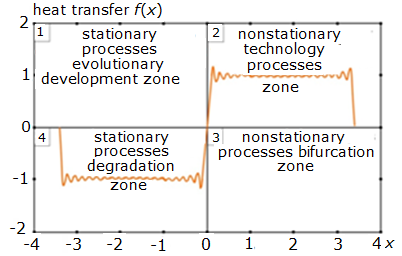Figure 1. Presentation of the negative effects of Gibbs and the types of zones of heat transfer processes with different speeds of implementation

It is proposed to consider the graphs of the initial function as a reflection of the energy efficiency of the heat transfer processes f(x) = Hi(x) of the burner device in the estimates of the impact indicators of the regulators of the automatic control system. An indicator property of improving Hi (x) of such a device is, for example, an increase in the efficiency of the boiler unit (thickened line). We propose interpreting five successive approximations of the function (a larger number of them can be shown) as mappings of successive extensions of the flare zone of fuel combustion, presented in the form of corresponding approximations of the efficiency functions of factor parameters (Figure 2). So the above addition of new properties of the improved element of heat engineering is shown, causing the expansion of the composition of the existing thermodynamic functions of the burner device. This requires additional functions of automatic control of the extended zone of the flare burning to control the corresponding parameters in the scheme for automatic control of combustion processes considered below.

Obviously, even with relatively small values when using iterative procedure (2), the graph of the approximating function approximates the initial function (1) quite well. Moreover, the approximating functions obtained using the proposed method are free from the disadvantages of expansion in Fourier series. The Gibbs effect is also completely absent. This means that the proposed approximating iterative procedure can interpret the cooling processes of a plate simulating the so-called thermally thick burner object under intense external cooling.

## 2.png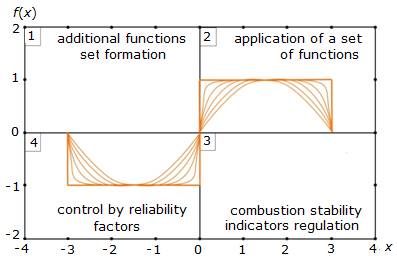Figure 2. Graphs of five consecutive approximations of the energy efficiency functions of the expansion of the flame combustion zone

The dependences in Figures 1 and 2 can be interpreted as bistable processes in the furnace of a boiler unit, characterized by an unsteady exponential increase in temperature during chemical reactions and overcoming the threshold barrier of heat supply at a certain point in time. This leads to a shift in the direction of the chemical reaction towards the formation of combustion products. Such processes are described by the equation:

$f(T)=C^{-1} q(T)-\xi\left(T-T_{1}\right)$,

where, Т=Т1 corresponds to the absence of combustion. That is, when determining the conditions for the occurrence of a flame and further stable combustion with a reduced consumption of the air-fuel mixture, it is necessary to consider the factors affecting the entropy of the system combining fuel, air, burner devices, the torch, and the enclosing structures of the combustion chamber of the boiler unit. The relationship between entropy and the time of exposure to the system is characterized according to I. Prigogin’s principle by the formula: $\frac{\Delta S}{\tau}=\left(\frac{\Delta S}{\tau}\right)_{\min }$.

According to it, the entropy is maximum when the system reaches an equilibrium state. However, this is hindered by external factors that contribute to the transition of the system to a stationary nonequilibrium state, which is characterized by the minimum value of the rate of occurrence of entropy under these conditions. This is proved by special calculations.

The existence of the Gibbs effect in approximating functions by trigonometric expressions also makes us critical of the proof of some important theorems, which reduces the accuracy of calculations. In particular, in the theory of signal transmission, the Kotelnikov's theorem, known in the English literature as the Nyquist theorem, is widely used. In the proof of the theorem V.A. Kotelnikov To approximate functions, it uses the so-called integral sine defined by the expression.

$Si(x)=\int\limits_{0}^{x}{\frac{\sin t}{t}dt}$    (4)

Based on the integral sine V.A. Kotelnikov builds a function $Si(T(\omega +{{\omega }_{1}}))-Si(T(\omega -{{\omega }_{1}}))$ to prove the theorem, where $\omega$ is argument, $T,\ {{\omega }_{1}}$ are some parameters. Moreover, he claims that with increasing $T$ this function tends to the limits shown in Figure 3a, and is equal to: zero for $\omega >{{\omega }_{1}}$; $\pi$ for $\omega <{{\omega }_{1}}$.

## 3.png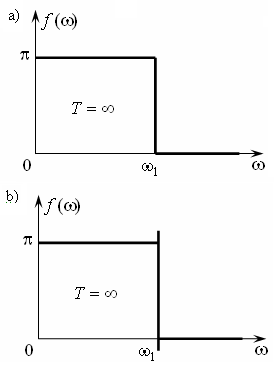Figure 3. Graphs of the limit function in the Kotelnikov's theorem (a) and S.V. Alyukov's refinements (b) to correct the control mechanism

Alyukov proved that the graph of the limit function will have the form shown in Figure 3b. That is, for any, even arbitrarily large, but finite values of the parameter, there are always those for which the values constructed by V.A. Kotelnikov functions will be different from, and there will always be those for which its values will be nonzero. Moreover, it is important to note that this difference with the increase does not tend to zero, but to a certain number other than zero, approximately equal to 0.281 . In this study, we propose to take this into account when correcting approximations of the functions of the regulatory influences of an automatic control system for combustion processes in an extended zone. With the consent of the scientific community and confirmation of the evidence, it is proposed to call the indicated number the Alyukov constant (in Latin transcription - CA).

The expansion of the torch combustion zone brings new opportunities to increase the energy efficiency of boiler units and determines the need for additional functions and control indicators. Therefore, additional time and resources are required to account for the new regulatory parameters. Proposed S.V. Alyukov mathematical tools for approximating functions will expand the technical and economic capabilities of more precise regulation of processes of spasmodic form.

The expansion of function (2) in the Fourier series has all the disadvantages described above. To eliminate them, it is proposed to approximate the initial step function by a sequence of recursive periodic functions

$\left\{f_{n}(x) | \begin{array}{l}f_{n}(x)=\sin \left((\pi / 2) \cdot f_{n-1}(x)\right) \\ f_{1}(x)=\sin x ; n-1 \in N\end{array}\right\} \subset$

$\subset C^{\infty}[-\pi, \pi]$     (5)

Let $\left\{ {{f}_{n}}(x) \right\}\subset {{L}_{2}}[0,\pi /2]$ and ${{f}_{0}}(x)\in {{L}_{2}}[0,\pi /2]$. Since $\underset{n\in N}{\mathop{\sup }}\,\underset{x\in [0,\ \pi /2]}{\mathop{\sup }}\,\left| {{\text{f}}_{n}}(\text{x}) \right|=1<\infty$ (due to the boundedness of the functions${{f}_{n}}(x)$) and $\underset{n\in N}{\mathop{\sup }}\,\underset{0}{\overset{\pi /2}{\mathop{\text{Var}}}}\,{{\text{f}}_{\text{n}}}=1<\infty$ (due to the monotonicity of the functions ${{f}_{n}}(x)$on the interval $[0,\pi /2]$), on the basis of Helly's theorem in the sequence $\left\{ {{f}_{n}}(x) \right\}$ we can extract a subsequence converging at each point $[0,\ \pi /2]$ to some function $f$,  moreover $\underset{0}{\overset{\pi /2}{\mathop{Var}}}\,f\le \underset{n\to \infty }{\overset{\_\_\_}{\mathop{\lim }}}\,\underset{0}{\overset{\pi /2}{\mathop{Var}}}\,{{f}_{n}}$. We show that the initial function $f$ can act as such a function${{f}_{0}}(x)$ .

5. Description of Heat and Mass Transfer Processes in the Mechanism for Regulating the Energy Efficiency of Heat Engineering Elements

Theorem 1. A sequence of functions ${{f}_{n}}(x)$ converges to the initial function ${{f}_{0}}(x)$), with the convergence being point -by-point, though not uniform.

Theorem 2. The sequence of approximating functions ${{f}_{n}}(x)$ converges along the norm towards the initial function ${{f}_{0}}(x)$ in Banach ${{L}_{1}}[0,\pi /2]$ and Hilbert spaces of measurable functions ${{L}_{2}}[0,\pi /2]$.

The proofs of the theorems are given in the paper .

The sequence of approximating functions ${{\text{f}}_{\text{n}}}(\text{x})$ in spaces ${{\text{L}}_{1}}[-\pi ,\pi ]$ and ${{\text{L}}_{2}}[-\pi ,\pi ]$is fundamental, whereas the sequence ${{\text{f}}_{\text{n}}}(\text{x})$ is not fundamental in the space $\text{C}[-\pi ,\pi ]$.

The features of the spaces and characteristics of the processes taken into account in the above evidence allow us to draw conclusions about their applicability for modeling the studied heat and power processes. In the sequence of approximating functions (2), a number $\pi /2$ was used as a constant factor, but it is possible to take another factor, which can be variable. Instead of a sine, the proposed approximation method can use cosine and other trigonometric functions, as well as their combinations. For example, using the sequence of recursive functions below

\left\{ {{f}_{n}}(x)\ \left| \begin{align} & \ {{f}_{n}}(x)=\cos (\varphi {}_{n}(x)),\ {{\varphi }_{n}}(x)= \\ & =(\pi /2)\cdot \sin ({{\varphi }_{n-1}}(x)),\ {{\varphi }_{1}}(x)= \\ & =x,\ n-1\in N \\\end{align} \right. \right\}\subset {{}^{\infty }}[-\pi ,\pi ],

We may approximate short-term impulses. These functions can be used for mathematical models describing the transmission of short-term signals, mechanical and heat power systems with shock interactions, etc.

Note that, despite the impulse (hence, essentially nonlinear) form of the graphs of such functions, they are continuous analytical functions and allow the use of analytical methods. The approximation error in this case in spaces ${{\text{L}}_{1}}[-\pi ,\pi ]$ and ${{\text{L}}_{2}}[-\pi ,\pi ]$ can be arbitrarily small.

Additional possibilities for approximating spasmodic processes are provided by the use of the method of generalized functions . They are significantly different from ordinary functions. An example is the delta function or the Dirac function. To establish a connection between the abstract mathematical concepts of generalized functions and the real meaning of modeling processes, one can use approximations of these functions in order to model jump processes. The article proposes new methods that allow us to understand the internal structure of generalized functions, their derivatives, and features of their use in the power system. It is known that the delta function is determined by the functional by the expression

\delta \text{(}x\text{)}=\left\{ \begin{align} & +\infty ,\ x=0,\ \\ & 0,\ \forall x\ne 0, \\\end{align} \right.    (6)

moreover $\int\limits_{-\infty }^{+\infty }{\delta \text{(}x\text{)}dx=1.}$

We introduce a sequence of step functions of the form

{{\delta }_{n}}(x)=\left\{ \begin{align} & n/2,\ \forall x\in [-1/n,\ 1/n], \\ & 0,\ \forall x\notin [-1/n,\ 1/n]. \\\end{align} \right.    (7)

It is easy to see that for any figure under the graph of such a step function is equal to unity. Find the values of the coefficients of the Fourier series on the segment $\text{ }\!\![\!\!\text{ }-\pi ,\ \pi \text{ }\!\!]\!\!\text{ }$

${{a}_{0}}=\frac{1}{\pi }\int\limits_{-\pi }^{+\pi }{{{\delta }_{n}}(x)dx=}\frac{1}{\pi }\int\limits_{-1/n}^{1/n}{\frac{n}{2}dx=}\frac{1}{\pi };$

${{a}_{k}}=0,$due to the parity of the function;

\begin{align} & {{b}_{k}}=\frac{1}{\pi }\int\limits_{-\pi }^{\pi }{{{\delta }_{n}}(x)\cos kxdx}= \\ & =\frac{1}{\pi }\int\limits_{-1/n}^{1/n}{\frac{n}{2}\cos kxdx=\frac{1}{\pi }\cdot \frac{n}{2}\cdot \frac{2}{n}\cos (k{{x}^{*}})=\frac{\cos (k{{x}^{*}})}{\pi }},\ \\ & {{x}^{*}}\in [-1/n,\ 1/n], \\\end{align}

by virtue of the mean value theorem of a certain integral.

Since the delta function $\delta (x)=\underset{n\to \infty }{\mathop{lim}}\,{{\delta }_{n}}(x)$and, noting that, ${{x}^{*}}\underset{n\to \infty }{\mathop{\to }}\,0$ we find ${{b}_{k}}=\frac{1}{\pi }$. Therefore, the expansion of the delta function in a Fourier series on the interval$[-\pi ,\ \pi ]$ has the form $\delta (x)=\frac{1}{2\pi }+\frac{1}{\pi }\sum\limits_{k=1}^{\infty }{\cos (kx).}$

For a finite series, we have an approximate relation

$\delta (x)\approx \frac{1}{2\pi }+\frac{1}{\pi }\sum\limits_{k=1}^{n}{\cos (kx).}$

The analysis shows that even with a significant number of harmonics (in our case), the approximation error is very large. This is the Gibbs effect . For example, note that the delta function is non-negative. The minimum value of the constructed approximation (Figure 2) is negative and amounts to - 69.182. Moreover, with an infinite increase in the number of terms in the approximating Fourier series, the minimum value of its sum tends to $-\infty$. This corresponds to the statement proved in articles [4, 5] about the possible infinitely large error in approximation using the Fourier series. In other words, the approximation by Fourier series even with an infinite number of terms does not correspond at all to the original delta function.

To construct such a sequence, we use the fact that the delta function is a derivative of the Heaviside function or the unit jump function, which is defined as follows

H(x)=\left\{ \begin{align} & 1,\ \forall x>0; \\ & 0,\ \forall x<0. \\\end{align} \right..

It is proposed to approximate the Heaviside function by a sequence of functions of the form ${{H}_{n}}(x)=0,5(1+{{f}_{n}}(x))$, where the sequence of recursive functions ${{f}_{n}}(x)$ is determined by the relation .

For example, Figure 4 shows graphs of three successive approximations that interpret the three above-mentioned expansion of the functions for increasing the energy efficiency of the heat transfer process Hi(x) of a new burner device while expanding the flare combustion zone depending on the increase in innovation factor (x) over time

${{H}_{9}}(x)=0,5(1+\sin (A(A(A(A(A(A(A(A(x))))))))))$, ${{H}_{10}}(x)=0,5(1+\sin (A(A(A(A(A(A(A(A(A(x)))))))))),$ ${{H}_{11}}(x)=0,5(1+\sin (A(A(A(A(A(A(A(A(A(A(x))))))))))),$ (8)

where, $A(x)=\frac{\pi }{2}\sin x$.

The thickness of the graph in Figure 4 increases as the number of the approximating dependence increases, but it is when using the 9,10 and 11 nested approximation functions that display the gradual expansion of the flare's burning zone, it becomes possible to achieve a maximum energy effect. This is shown below on the basis of mathematical justification.

## 4.png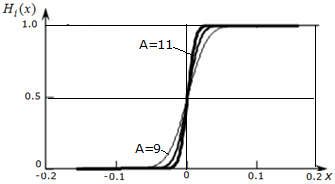Figure 4. Graphs of Heaviside function approximation with a sequential increase in the number of nested functions modeling the expansion of the combustion zone

A static representation of processes is sufficient for estimating only stationary processes (at t≤0) in cycle 1 in comparison with cycle 2 of non-stationary processes. This can be established by correlating the relevant criteria for energy efficiency. In final increments, they can be used to regulate the processes of the power system (the practice of regulation based on the relative increment of energy efficiency indicators is known):

$\left| {{H}_{i}}(x){{x}_{2t2}} \right|\ge \left| {{H}_{i}}(x){{x}_{1t1}} \right|$     (9)

The insufficiency of static estimates is obvious, characterizing mainly the zones of processes of evolutionary improvement of processes and elements of heat engineering. The zone of the revealed short-term spasmodic effect of the jump-transition to highly efficient processes (in the range of -0.05 ... 0.05 in Figure 5) needs to be studied on the basis of estimates of dynamic processes under strong factor impacts according to their intensity and speed in automatic control systems of combustion processes. For analysis, it is necessary to determine the parameters of speed, acceleration, and others, taking into account the characteristics of changes and control parameters in the estimates of the derivatives of the approximated functions of the system of equations (6). They will allow us to identify critical points of the singularity at x = 0 and others that determine the loss of stability of the system in the mathematical and technical sense.

We identified the new possibilities for improving the quality of control of the spasmodic development of complex systems, which we called the “stretching” effect of controlling the dynamics of heat transfer processes in the space of the expanded torch burning zone of the object under study. As a result of modeling, a zone of maximum effect of the integral application of the above complex of standard and additional functions of the burner device, interpreted by an increase in the number of embedded approximation functions from 9 to 11, was established. The established regularity also confirms the theoretical possibility and objectivity of the existence of the effect of conditional expansion of space and time of regulation of the indicator-property of energy efficiency in the expanded zone of combustion of the torch. The effect of "expanding" the space and time of decision-making for technical and socio-economic systems is justified by approximating the display of the jump-transition by a certain number of embedded functions in studies of Alabugin and Alyukov for any complex systems.

Finding the first derivatives of the approximations of the Heaviside function, we obtain successive approximations $\frac{d{{H}_{9}}(x)}{dx}$, $\frac{d{{H}_{10}}(x)}{dx}$ and $\frac{d{{H}_{11}}(x)}{dx}$ for the delta function. Their graphs are shown in Figure 5. With a sufficiently large number of nested functions, we obtain an approximating function $\frac{d{{H}_{18}}(x)}{dx}$, the graph of which is obtained using the MathCAD computer program and is shown in Figure 6. The practical inapplicability of such an interpretation and the lack of its economic efficiency are obvious.

Comparing the graphs, we note once again that the proposed approximation methods give a much more accurate approximation of the delta function than the Fourier series. Moreover, the accuracy of approximation can be increased to an arbitrarily large degree by increasing the number of nested functions. The height of the approximation peak (amplitude) can be determined by the integral condition in the definition of the delta function. It can interpret temperature gradients (during heat transfer) or mass concentration. Therefore, in the zone of the temperature field [- 0.15 ... 0.15] modeling a flat wall, one can analytically display the explicit form of the functions of the temperature field. It is proposed to consider as such the dependences of the studied indicator-property of the energy efficiency of heat transfer Hn or Hi on time x and a certain number (in this case, three) of embedded functions in the system of equations (6) that simulate the sequential expansion of the flare combustion zone and the use of additional functions of its automatic regulation in the boiler unit.

Differentiating the approximating functions of the considered sequence ${{H}_{n}}(x)=0,5(1+{{f}_{n}}(x))$, we obtain

$\frac{d{{H}_{n}}(x)}{dx}=\frac{{{\pi }^{n-1}}}{{{2}^{n}}}\prod\limits_{k=1}^{n-1}{\cos \ \left( \frac{\pi }{2}{{f}_{k}}(x) \right)}\cdot \cos \ x$     (10)

## 5.png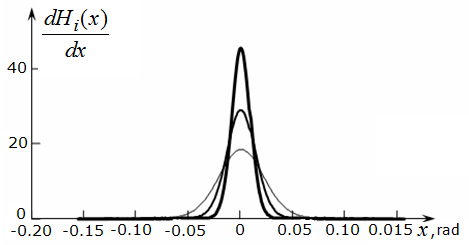Figure 5. Graphs of approximations of a delta function with an increase in the number of nested functions modeling the growth of energy efficiency when the combustion zone is expanded

## 6.png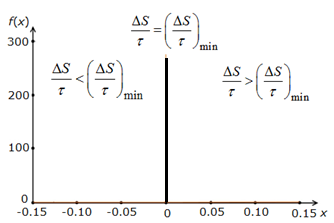Figure 6. The graph of the approximation of the delta function with an almost inexpedient number of nested functions according to the criterion of energy efficiency of the burner device

Substituting the obtained expression for the derivatives $x=0$, taking into account the parity of the $\delta$- function, we find the value for the peak height ${{}_{n}}$of the approximating functions as an indicator of the maximum heat transfer

${{A}_{n}}=\frac{{{\pi }^{n-1}}}{{{2}^{n}}}$     (11)

Taking into account the above justified Alyukov constant, the indicator-property Hi in assessing the jump-like development in zone 2 must be increased, and in zone 3, reduced by the indicated constant CA = Hк (when reducing them to the same dimension). Substituting the resulting expression for the derivatives $x=0$, we find the maximum corrected value of the function ${{H}_{i}}(x) ={{}_{n}}$ for zones 2 and 3:

${{A}_{n}}=\frac{{{\pi }^{n-1}}}{{{2}^{n}}}\pm CA.$     (12)

Since we approximated generalized functions by analytic functions, we can differentiate these approximating functions and find their derivatives of any order. Thus, we obtain approximations of derivatives of generalized functions with any degree of accuracy. For example, similarly to how this was done to approximate the delta function, we can plot the approximations of its derivatives. Figure 7 shows graphs of successive approximations of the second, third and forth derivatives of the Heaviside function (the first, second and third derivatives of the delta function).

## 7.png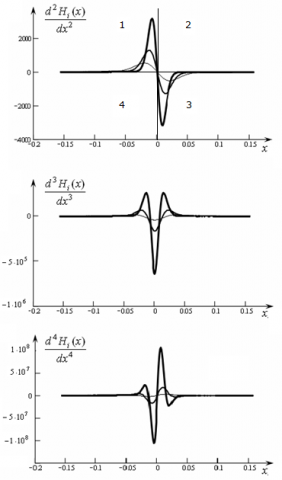Figure 7. Graphs of approximations of derivatives of the delta function for displaying four types of regulators of a heating element

The problem arises of interpreting derivatives in accordance with the specifics of the processes of thermodynamics and heat engineering. It should be borne in mind that the combination of such derivatives forms the organizational and technical tools for the mechanism for regulating the resulting indicator-property of energy efficiency of non-stationary heat transfer processes of the studied heat engineering element and heat power plant in the phase of high-tech organizational and innovative dynamics [20, 21]. The evolutionary, spasmodic, bifurcation, low-efficiency, degrading nature of heat transfer processes, of course, must comply with the second and other laws of thermodynamics [22-24]:

• evolutionary processes distinguish zone 1 of the control cycle at Hi≥0, x < 0. The negativity of time can be interpreted as stationary of heat transfer processes and increase of positive entropy;

•spasmodic non-stationary processes of increasing positive entropy are characteristic of zone 2 of the graphs of all derivatives for Hi≥0, x≥0. In this zone, the greatest results of increasing heat transfer are achievable;

• bifurcation non-stationary processes occur in zone 3 at Hi≤0, x≥0. They mean a decrease in positive entropy and a decrease in an indicator property;

•low energy efficiency indicators with an increase in entropy in zone 4 of stationary processes with Hi≤0, x≤0 require special regulatory influences.

All of the above determines the significance of the estimates of entropy in the development of measures to increase the energy efficiency of the element under study in the subsystem of the burner devices of the boiler unit . The uncertainty of the dynamics results determines the need to assess the probabilistic factors of the positive synergy of the regulatory impact of personnel and automation. To this end, the entropy of heat transfer processes can be determined using the well-known Shannon formula . This will allow us to establish the probabilistic indicators of the effects of the four regulators of the formation of series-chain effects that we propose on the energy efficiency of heat transfer processes in the burner subsystem:

In this study, it is proposed to interpret the above-defined complex of existing (in standard-type burner devices) and newly proposed additional functions [25, 26] for organizing processes to increase energy efficiency while expanding the flare zone with the above defined embedded functions for approximating spasmodic and evolutionary processes. The empirical method for analyzing the differences in the graphs of higher derivatives based on the approximation of the delta function made it possible to determine and specify the control actions of the automatic control system of combustion processes:

• zone 1 of the controller 4a of the rate of evolutionary processes of increasing energy efficiency in the assessment of heat transfer Hi according to indicators of taking into account the boundary conditions of the first kind, which determine the accuracy of regulation of dynamics. This type of regulator has (Figure 7) symmetrical, multidirectional and strong effects in zones 1 and 3 of the regulatory processes. Modeling based on the first derivative approximation of the energy efficiency function provides the possibility of estimating the rate of regulation of the fuel and air supply using standard control parameters of the investigated heat engineering facility;

• zone 2 of the controller 4b of the rate of a jump-like increase in energy efficiency by taking into account the boundary conditions of the second kind under the influence of symmetric, multidirectional and weak types on the results of the operation of controller 4a. Simulation based on the second derivative interprets high-tech or innovative acceleration of evolutionary heat transfer processes using new methods and technical means (frequency regulation used for blow fans);

• zone 3 of controller 4c, the rate of increase in energy efficiency in terms of boundary conditions of the third kind under the influence of asymmetric, multidirectional and strong types on controller 4b in all zones to stabilize the process with maximum heat transfer in zone 2. Modeling based on the third derivative interprets the processes of leveling negative bifurcation phenomena due to an increase in the uncertainty of changes in the level of heat transfer efficiency in zone 3;

• zone 4 of controller 4d (displays additional protection to increase reliability, Figure 7) of the rate of increase in energy efficiency according to indicators of boundary conditions of the fourth kind under the influence of asymmetric, multidirectional and weak types in all zones on the results of the operation of controller 4c. Explanations for Figure 7 are as follows: Вr is fuel consumption, nspp  is speed of the solid fuel conveyor belt, Kfj, Ktj are correction factors affecting the determination of the optimal flame temperature Тfi and the temperature of the combustion products at the outlet of the furnace Тt"i, which are compared with standard values of these temperatures Тfjn, Тt"in. Regulation becomes possible in the presence of a propagating combustion wave depending on the temperature and concentration of the reacting substances μ: f(T,μ)=C-1q(T,μ) – ξ(T-T1). Thus, the alleged analogies of the energy efficiency function and the non-stationary combustion process become apparent. The first derivative of this function is shown in the block diagram (Figure 8) and is associated with the choice of the type of fuel, its concentration, as well as the load of the boiler unit. The second derivative is determined by the type of high-tech burner devices b. The third reduces the role of deviations of the absolute temperature of the flame and combustion products at the outlet of the furnace c. The fourth eliminates emergency situations during the operation of the boiler unit d.

## 8.png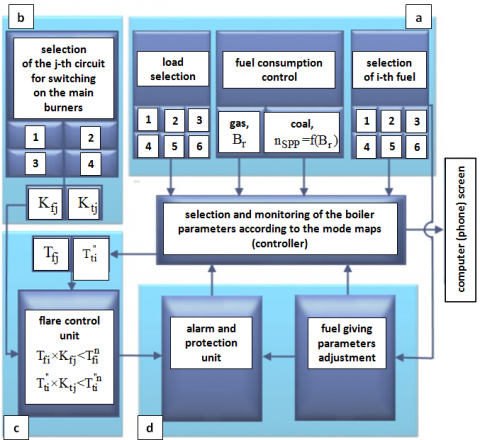Figure 8. The scheme of combustion automatic control

The simulation based on the fourth derivative displays the processes of minor adjustment of the regulatory subsystem to obtain more accurate solutions in the conditions of spontaneous processes of increasing heat transfer (Figure 8). Successive actions of the system of regulators in an interconnected circuit and control loop with direct and feedback connections provide new properties of the energy-efficient operation of the object under study. The diversity of the regulatory parameters taken into account necessitates a transition to a three-dimensional space for measuring interconnections, an increase in the number of considered factors of the boundary conditions, and leads to an increase in the multifactorial nature and complexity of the functional and probabilistic effects on the indicator-property under study.

6. Results of Applying the Methodology of Combining Methods and Regulating the Heat and Mass Transfer Mechanism

Simulations were performed using the standard k-omega model. The authors also propose to use in the calculations the original version of the approximation of the generalized functions used in the simulation, which are derived from the delta-function. This is relevant, for example, when determining the properties of coal dust fractional separation functions. The complexity of using these functions is reduced to the methods of their approximation, which are best known in Engineering:

$\mathrm{F}_{\min }\left(\delta_{i}\right)=1-\frac{\mathrm{p}}{\sqrt{2 \pi}} \int_{-\infty}^{t} \exp \left(-\frac{\mathrm{p}^{2}}{2} \ln ^{2} \mathrm{t}\right) \mathrm{dt}$     (13)

where, $\mathrm{t}=\delta_{\mathrm{i}} / \delta_{50}, \delta_{50}$ represents the separation boundary, that is, the size of the fraction that corresponds to the distribution of particles equally into large and small fractions; p is the coefficient that characterizes the steepness of the curve. This distribution (13) is called normal-logarithmic.

At the same time, the author of the paper Alyukov S.V. developed new methods of approximation of generalized functions.

The delta function is a derivative of the Heaviside function or the unit jump function, which is defined as

H(x)=\left\{ \begin{align} & 1,\ \forall x>0; \\ & 0,\ \forall x<0. \\\end{align} \right.\,     (14)

Alyukov S.V. suggested to approximate the Heaviside function by a sequence of functions of the form ${{H}_{n}}(x)=0.5(1+{{f}_{n}}(x))$, where the sequence of recursive functions is determined by the relation

$\left\{f_{n}(x) | \begin{array}{l}f_{n}(x)=\sin \left((\pi / 2) \cdot f_{n-1}(x)\right) \\ f_{1}(x)=\sin x ; n-1 \in N\end{array}\right\} \subset$

$\subset C^{\infty}[-\pi / 2, \pi / 2]$     (15)

Finding the first derivatives of the Heaviside function approximations, we obtain successive approximations for the delta function. Example of approximation of the second derivative of the original delta-function is shown in Figure 9.

Since we approximated the generalized functions by analytic functions, we can differentiate these approximating functions and find their derivatives of any order. Thus, the main functions of the parameters for k-omega computer simulation of air flow can be approximated by the new method, not previously used in world practice.

The obtained approximations more accurately describe the original function. The suggestions for improving the mathematical apparatus of the standard k-omega model will help researchers to develop the theory of approximation by the recursive functions of Dr. S.V. Alyukov. The results will solve one of the important problems of modeling at this stage of its development, namely reducing the calculation time and the suitability of the constructed model for similar installations and devices.

## 9.png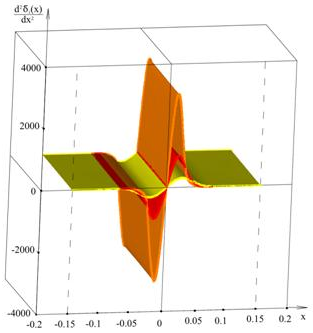Figure 9. Example of approximation of the second derivative of the original delta-function

As a result (Figure 10), it was obtained that with the help of new methods of approximation and analysis of deviations of the air flow rate in the channel, it is possible to obtain more accurate results of experimental and numerical studies. In particular, the following is the 3D distribution of deviations in the calculation of air velocity.

## 10.png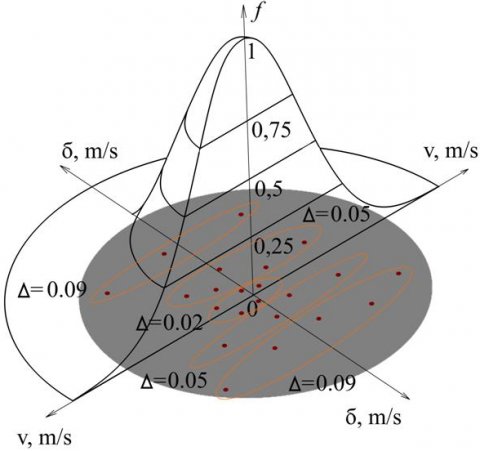Figure 10. Results of new method applying

The measurement results are affected by a large number of sources of small random errors. The whole set of measurements has a symmetric bell-shaped Gauss function as a limit distribution. The distribution center, which coincides with its maximum, will be the true value of the measured value. When using the method of approximation of Dr. S.V. Alyukov, which is considered for the first time in relation to numerical methods of gas dynamics and experimental data for the flow of coal and air in the aerodynamic channel.

7. Computational Modeling

The values of the velocity of air and coal obtained by ANSYS (Figures 11 and 12) illustrate that the local flow velocity increases with temperature. The velocity of the outlet of burner, which is the highest velocity, it is around 8.14 m/s. In the course of mathematical modeling of the combustion process, a solid-state three-dimensional model of the channel of the burner device was built, a grid was applied to it (Figure 11).

## 11.jpg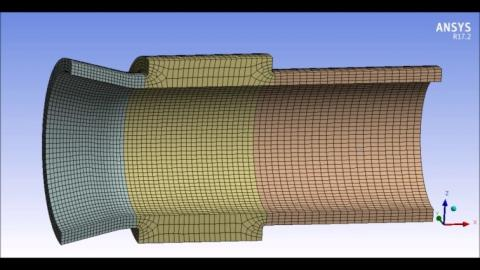Figure 11. Mesh superimposed on the solid state model of the burner

The flow of a mixture of fuel and air was considered in two cases, namely with an oxygen content of 16 and 12 percent. This condition was necessary for a complete simulation of the mixing process and chemical reactions at different distances from the cut of the burner, since the burners are located in two tiers on top of a real boiler unit. Thus, on the lower tier, the condition is fulfilled under which the fuel burns out immediately and completely with an oxygen content of 16 percent in the air. At the same time, on the second tier, at a certain distance from the cut of the lower burners, oxygen is gradually consumed, and its content approaches 12 percent.

In a real boiler plant, the degree of fuel burnup can be estimated not only by the oxygen content, but also by the presence of CO in the combustion products. Actually, the higher the CO content, the lower the degree of burning of coal dust, and the worse the process of burning organic fuel in the furnace of a boiler unit is organized. As a result of the mathematical modeling of the combustion process of two types of coal dust, approximately equivalent temperature fields were obtained, which are shown in Figures 12 and 13. Figure 12 shows the temperature fields for the lower tier of burner devices with an oxygen concentration of 16 percent.

Moreover, the speed at the outlet of the burner was, as mentioned earlier, approximately 8.14 m/s. In the core of the torch, the maximum absolute temperature reached 1773 K. But, as the experience of operating boiler units shows, the most significant for researchers is not the maximum absolute temperature, but the temperature at the end of the intense combustion zone Tf. This temperature averaged 1454 K, which is clearly seen in Figure 13. The values of Tf and velocity v are listed in Table. The average characteristics of the Tash-Kumyr and Kavak coals, which are most often used at the thermal power plants of the city of Bishkek, were used in the simulation. These coals are local, can be used in a mixture with each other, or burned separately. But, since the authors have experimental data obtained for a mixture of coals, it makes sense to use the characteristics of a mixture of coals in the simulation.

## 12.pngFigure 12. Results of computational modeling (concentration of oxygen is 16 percent)

## 13.png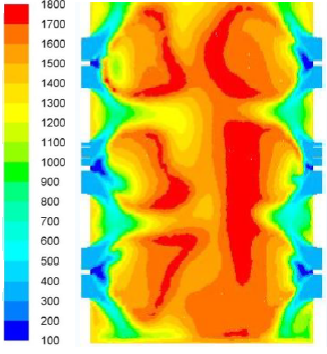Figure 13. Results of computational modeling (concentration of oxygen is 12 percent)

In addition, the authors give the trajectories of the movement of coal particles in the furnace of the boiler unit in Figure 14. From this figure, conclusions can be drawn about further improvement of the combustion processes and the supply of the mixture of coal particles and air through two tiers of burners into the furnace of the boiler unit.

## 14.png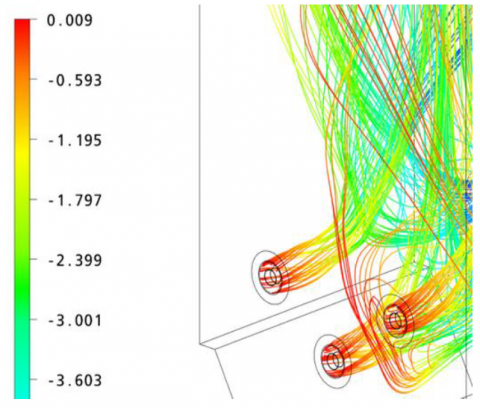Figure 14. Results of computational modeling (velocity distribution, particle trajectories in the furnace of the boiler unit)

8. Experimental Data

Experimental data were collected on the boilers of the Bishkek thermal power plant with the participation of specialists from the Ministry of energy of the Republic of Kyrgyzstan. Figure 15 shows a photo of burning dust Kovak coal .

## 15.pngFigure 15. Picture of experiment investigation

The experiments were carried out using thermocouples, thermal probes and pyrometers. All devices are certified. In addition, instruments for determining the concentration of substances in gases were used to determine the oxygen and CO content. To determine the degree of fuel burnout, tubes were used for sampling unburned coal dust from the torch in the furnace of the boiler unit.

9. Verification

Now we can compare the results and mathematical calculations with the experimental data of the steam generator in Table 1.

The authors emphasize that the obtained simulation results in ANSYS are very similar to theoretical and experimental data. The differences are insignificant, since the error in experimental measurements was leveled by the use of modern instruments certified by the European Union. As mentioned earlier, the error of the proposed calculation method using approximating functions instead of Fourier series makes the theoretical calculation more correlated with respect to the data obtained during the experiments.

The error in mathematical modeling was reduced due to the use of specific grids and models used only for a heterogeneous mixture, namely a mixture of coal particles with air. For example, this is clearly seen in the values of the velocity v. In a theoretical calculation without approximation, the velocity value ranged from 8.15-8.17 m/s, that is, on average it was 8.16 m/s. At the same time, when using approximating functions, the velocity value according to theoretical calculations was 8.12 m/s, Table. This value is the closest to the experimentally obtained values of 8.08 m / s. Thus, the greatest differences are between the experimental data and computer simulation data, while the theoretical calculation showed the average values between the simulation and the experiment.

Table 1. Comparison of results of Kavak coal

 Parameter Theoretical calculations BKZ steam generator CFD analysis Velocity (v) m/s 8.12 8.08 8.14 Temperature (Tf) K 1451 1446 1454

According to the results of this research, it can be concluded that the temperature of the steam generator flame is actually matches to the theoretical results as demonstrated.

It should be noted that the results were verified for specific conditions of fuel combustion in the furnace of the boiler unit BKZ CHP in Bishkek. However, as experience with the use of approximating functions has shown, they can be used for other similar calculations of heat exchange equipment. In particular, the results can be transferred to boiler units of similar designs.

10. Conclusions

Thus, the article discloses the results of a scientific study of methods for analyzing thermodynamic processes, substantiates their differences from existing theoretical, methodological and methodological approaches, identifies new practical possibilities for regulating heat transfer processes, concretized as follows:

1. The methodological approach to implementing the conception of combining the capabilities of existing theoretical models of organizational and mathematical research is justified to expand the capabilities of analytical methods for displaying heat transfer processes in heat engineering elements. The laws, criteria, and indicators of specifying calculations of unsteady heat transfer processes as applied to burner devices with an extended flare zone are studied. The methods are based on the principles of theories of combustion and heat transfer, which include a new set of functions and indicators of the combustion process. The dependences of the energy efficiency of the element under study and the factor indicators and regulation parameters of heat transfer processes (design features of the burners that affect the processes in the ignition and active combustion zones) are identified and refined. The conceptual category “combustion zone” has been clarified taking into account the peculiarities of the processes in the expanded torch burning zone. Based on the author’s indicator of a generalized performance assessment, constructed using the methods of thermodynamics and solving systems of nonlinear differential equations, the possibilities of assessing the expected growth of the indicator are determined. This allows you to increase the validity and accuracy of calculations in the design projects of high-tech elements of heat engineering.

2. Based on the analysis of existing methods, the conceptual apparatus has been refined and supplemented, including an expanded set of control functions and regulators, which can be used in automated control systems, taken into account in modernized burner designs that support a more reliable boiler operation mode. Such a toolkit is distinguished by a generalization of several basic factors affecting the combustion process. This allows you to determine the main directions and methods for improving the processes and elements of burner devices and auxiliary boiler equipment.

3. The influence of changing paradigms in the development of complex systems without taking into account the new possibilities of combining theories and methods of different branches of science and technology on the practice of applying methodologies that take into account integration-convergent and organizational-technological functional features of modeling and design of heating elements has been revealed. The principles of improving the analysis and regulation of evolutionary and spasmodic processes of improving the methodological foundations of the design of boiler units are formed.

4. Based on the integration of thermodynamic and mathematical methods, new possibilities are substantiated for expanding the space and time of regulation of combustion processes on the basis of a set of modernized numerical-analytical methods for modeling solutions to boundary-value problems of heat transfer in boiler units. To this end, additional functions and indicators for regulating processes in the expanded torch zone of the boiler unit have been developed, justifying tools for regulating the supply of fuel and air when using automated systems to ensure temperature stability in the active combustion zone and at the outlet of the furnace of the boiler unit.

5. The differences in the proposed methods for modeling combustion and heat transfer processes consist in the fact that it is proposed to use stepwise and generalized functions for interpreting non-stationary processes even in the case of multilayer models of control processes and monitoring systems for controlling boiler units, which allow to increase the accuracy of analytical solutions in comparison with Heaviside asymmetric unit functions. The zones of maximum energy efficiency corresponding to a certain number of embedded approximation functions and their derivatives for the formation of a process control mechanism are revealed.

6. The necessity of methods of static and dynamic research of the zone of maximum energy efficiency, modeled by a certain number of special approximation functions of the Dirac function, is substantiated. Based on them, the possibilities of interpreting temperature gradients during heat transfer through a flat wall and modeling the dependence of the energy efficiency of the process by a certain number of embedded approximation functions are determined. This reflects the sequential expansion of the flare combustion zone, measured by special indicators-factors as parameters of automatic control and regulation of the process under study.

7. An empirical method has been developed for analyzing higher order derivatives of the approximation of the delta function to model the control actions of the regulators of the automatic control system of combustion processes. The use of types of derivatives will allow the interpretation of different types of effects.

Acknowledgment

The work was supported by the Government of the Russian Federation (Resolution No. 211 of 16 March 2013), contract No. 02.A03.21.0011.

References

 Alabugin, A.A. (2017). Methodology of managing the integration of intellectual, research and investment resources to improve the efficiency of neo-industrial technological development of systems. Intelligence, Innovation, Investment, 4: 4-11.

 Alabugin, A.A. (2005). Managing the balanced development of the enterprise in a dynamic environment. Book 1. Methodology and theory of the formation of the adaptive mechanism of enterprise development management: Monograph. Chelyabinsk: Publishing House of SUSU, 362 p.

 Helmberg, G. (1994). The Gibbs phenomenon for Fourier interpolation. Journal of Approximation Theory, 78(1): 41-63. https://doi.org/10.1006/jath.1994.1059

 Meltzer, D. (1986). On the expressibility of piecewise linear continuous functions as the difference of two piecewise linear convex functions. Math. Program Study, 29:118-134. https://doi.org/10.1007/BFb0121142

 Vorontsov-Velyaminov, B.A. (1985). Laplace. Nauka, Moscow, 288 p.

 Ewald, W.B. (ed). (1996). Elements of Dynamic. From Kant to Hilbert: A Source Book in the Foundations of Mathematics Vol. 2, Oxford University Press.

 Osintsev, K.V. (2012). Studying flame combustion of coal-water slurries in the furnaces of power-generating boilers. Thermal Engineering, 59(6): 439-445. https://doi.org/10.1134/S0040601512060079

 Kijo-Kleczkowska, A. (2012). Research on coal-water fuel combustion in a circulating fluidized bed. Archives of Mining Sciences, 57(1): 79-92. https://doi.org/10.2478/v10267-012-0006-5

 Aidarova, S., Bekturganova, N,, Kerimkulova, M., Sharipova, A. (2014). The influence of surfactants to the stability of coal water suspension. Periodica Polytechnica Chemical Engineering, 58(Supplement): 21-26. https://doi.org/10.3311/PPch.7300

 Kijo-Kleczkowska, A. (2011). Combustion of coal–water suspensions. Fuel, 90(2): 865-877. https://doi.org/10.1016/j.fuel.2010.10.034

 Glushkov, D.O., Strizhak, P.A. (2017). Ignition of composite liquid fuel droplets based on coal and oil processing waste by heated air flow. Journal of Cleaner Production, 165: 1445-1461. https://doi.org/10.1016/j.jclepro.2017.07.207

 Zhou, M.S., Pan, B., Yang, D.J., Lou, H.M., Qiu, X.Q. (2010). Rheological behavior investigation of concentrated coal-water suspension. Journal of Dispersion Science and Technology, 31(6): 838-843. https://doi.org/10.1080/01932690903333788

 Staron, A., Kowalski, Z., Staron, P., Banach, M. (2014). Properties of coal-water suspensions. Przemysl Chemiczny, 93(5): 748-751. https://doi.org/10.12916/przemchem.2014.748

 Redkina, N.I., Khodakov, G.S., Gorlo, E.G. (2009). Rheology of coal-water slurries. Solid Fuel Chemistry, 43(6): 341-350. https://doi.org/10.3103/S0361521909060020

 Mohapatra, S., Kumar, S., Chakravarty, A. (2014). Transportation performance of highly concentrated coal-water slurries prepared from Indian coals. Applied Mechanics and Materials, 592-594: 869-873. https://doi.org/10.4028/www.scientific.net/AMM.592-594.869

 Alyukov, S.V. (2011). Approximation of step functions in problems of mathematical modeling. Mathematical Models and Computer Simulations, 3: 611. https://doi.org/10.1134/S2070048211050036

 Wang, H., Guo, S., Yang, L., Guo, Y., Jiang, X., Wu, S. (2015). Surface morphology and porosity evolution of CWS spheres from a bench-scale fluidized bed. Energy & Fuels, 29(5): 3428-3437. https://doi.org/10.1021/ef502923t

 Hatami, M., Bayareh, M. (2019). Numerical simulation of heat transfer from three-dimensional model of human head in different environmental conditions. International Journal of Heat and Technology, 37(3): 803-810. https://doi.org/10.18280/ijht.370317

 Alyukov, S.V. (2013). Approximation of generalized functions and their derivatives. Questions of atomic science and technology. Ser. Mathematical Modeling of Physical Processes, 2: 57-62.

 Giuliano, A., Catizzone, E., Barisano, D., Nanna, F., Villone, A., De Bari, I., Cornacchia, G., Braccio, G. (2019). Towards methanol economy: A techno-environmental assessment for a bio-methanol OFMSW/biomass/carbon capture-based integrated plant. International Journal of Heat and Technology, 37(3): 665-674. https://doi.org/10.18280/ijht.370301

 Dwivedi, K.K., Karmakar, M.K., Pramanick, A.K., Chatterjee, P.K. (2019). A brief review on hydrodynamic behaviour analysis of coal gasification in a circulating fluidized bed gasifier. International Journal of Heat and Technology, 37(3): 792-802. https://doi.org/10.18280/ijht.370316

 Valiullin, T.R, Vershinina, K.Y, Lyrshchikov, S.Y, Shevyrev S.A. (2017). Ignition of fuel based on filter cake. Coke and Chemistry, 60(3): 127-132. https://doi.org/10.3103/S1068364X17030073

 Toropov, E.V., Osintsev, K.V., Alyukov, S.V. (2018). Analysis of the calculated and experimental dependencies of the combustion of coal dust on the basis of a new methodological base of theoretical studies of heat exchange processes. International Journal of Heat and Technology, 36(4): 1240-1248. https://doi.org/10.18280/ijht.360411

 Alabugin, A.A., Alykov, S.V., Alabugina, R.A. (2016). Managing cooperation between business and university: Quality assurance using sustainable development, knowledge management system. International Confererence of Education, Research and Innovation, November 14th-16th, Seville, Spain. Published by IATED Academy, pp. 6938-6945. https://doi.org/10.21125/iceri.2016.0059

 Sharkov, O.V., Zolotov, I.A. (2011). Influence of the geometric properties of an external housing on its stress-strain state. Russian Engineering Research, 31(4): 335-337. https://doi.org/10.3103/S1068798X11040228

 Sharkov, O.V., Koryagin, S.I. (2017). Operational reliability of free-wheel mechanisms in a pulsed variable-speed drive. Russian Engineering Research, 37(1): 9-12. https://doi.org/10.3103/S1068798X1701021X